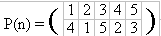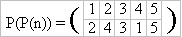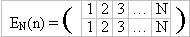Online JudgeProblem SetAuthorsOnline ContestsUser
Web Board
F.A.Qs
Statistical Charts
Problems
Submit Problem
Online Status
Prob.ID:
Register
Authors ranklist
Current Contest
Past Contests
Scheduled Contests
Award Contest
Register
Language:
Permutations
 Time Limit: 1000MS Memory Limit: 65536K Total Submissions: 3799 Accepted: 2080

Description

We remind that the permutation of some final set is a one-to-one mapping of the set onto itself. Less formally, that is a way to reorder elements of the set. For example, one can define a permutation of the set {1,2,3,4,5} as follows:This record defines a permutation P as follows: P(1) = 4, P(2) = 1, P(3) = 5, etc.
What is the value of the expression P(P(1))? It’s clear, that P(P(1)) = P(4) = 2. And P(P(3)) = P(5) = 3. One can easily see that if P(n) is a permutation then P(P(n)) is a permutation as well. In our example (believe us)It is natural to denote this permutation by P2(n) = P(P(n)). In a general form the defenition is as follows: P(n) = P1(n), Pk(n) = P(Pk-1(n)). Among the permutations there is a very important one — that moves nothing:It is clear that for every k the following relation is satisfied: (EN)k = EN. The following less trivial statement is correct (we won't prove it here, you may prove it yourself incidentally): Let P(n) be some permutation of an N elements set. Then there exists a natural number k, that Pk = EN. The least natural k such that Pk = EN is called an order of the permutation P.
The problem that your program should solve is formulated now in a very simple manner: "Given a permutation find its order."

Input

In the first line of the standard input an only natural number N (1 <= N <= 1000) is contained, that is a number of elements in the set that is rearranged by this permutation. In the second line there are N natural numbers of the range from 1 up to N, separated by a space, that define a permutation — the numbers P(1), P(2),…, P(N).

Output

You should write an only natural number to the standard output, that is an order of the permutation. You may consider that an answer shouldn't exceed 109.

Sample Input

```5
4 1 5 2 3
```

Sample Output

`6`

Source

[Submit]   [Go Back]   [Status]   [Discuss]Home PageGo BackTo top